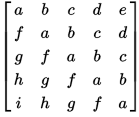365. Check if a matrix is a Toeplitz matrix

0

Medium

Determine whether a given `M x N` matrix is a Toeplitz matrix. A Toeplitz matrix, also known as a diagonal-constant matrix, is a matrix in which each descending diagonal from left to right is constant.

A matrix `Mat` of size `N x M` is considered a Toeplitz matrix if `Mat(i, j) = Mat(i+1, j+1) = Mat(i+2, j+2)` and so on. Here, `Mat(i, j)` refers to the element `Mat[i][j]` in the matrix.

For example, the following matrix is a Toeplitz matrix:Input Format

Two integers `M` and `N` representing the number of rows and columns in the matrix.
`N x N` integers representing the elements of the square matrix.

Output Format

true or false

Example

Input

4 5 3 7 0 9 8 5 3 7 0 9 6 5 3 7 0 4 6 5 3 7

Output

true

Constraints

0 <= M, N <= 10 0 <= mat[i][j] <= 100